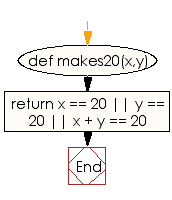﻿ Ruby Basic exercises: Check two integers and return true if one of them is 20 otherwise return their sum - w3resource# Ruby Basic Exercises: Check two integers and return true if one of them is 20 otherwise return their sum

## Ruby Basic: Exercise-19 with Solution

Write a Ruby program to check two integers and return true if one of them is 20 otherwise return their sum.

Ruby Code:

``````def makes20(x,y)
return x == 20 || y == 20 || x + y == 20
end

print makes20(10, 10),"\n"
print makes20(40, 10),"\n"
print makes20(15, 20)
``````

Output:

```true
false
true
```

Flowchart:Ruby Code Editor: